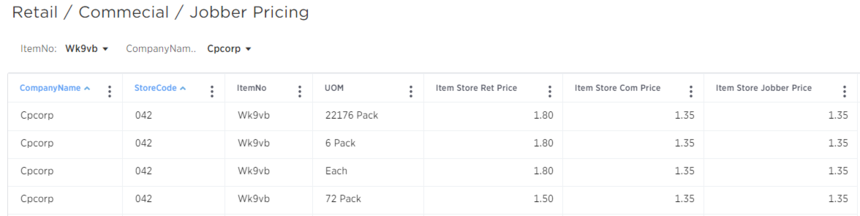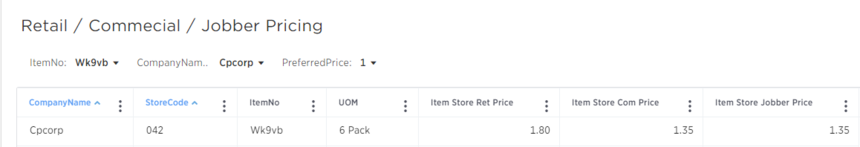# Using indicators in a formula rather than as a filter

I have a Price/UOM attribute where 1 = preferred and 0 = not preferred. This is used to determine which Price/UOM is used in instances where we have multiple Prices/UOMs available.  I can easily set a filter on an answer to only show when this is a 1 but I am looking to build this into an existing formula within a worksheet with other attributes and measures but I am encountering errors.  Is there a way to add this "filter" of =1 into a formula or is there a better option?  Just adding an if statement where preferred price = 1 does not work because it then follows the rest of the formula rather than removing the instances of 0 altogether.

6replies Oldest first
• Oldest first
• Popular•Jason  - you'll have to use the if(x=1) then 1 else 0 function. If you get an aggregation error try sum(if(x=1) then 1 else 0). Try it out and let me know if you need more help. Here's an example I'm working with:

unique count (if (sales > 100)) then account else 0). This gives me the count distinct of accounts with sales greater than 100.

Like
•Steph Fisher - Thanks Steph but I already tried that and it doesn't give me what I am looking for.  If I were doing a count, this would work... Here's the issue...

I want the data to be removed from the answer completely rather than just showing a 0 or blank.  For example, we have a product that comes in eaches, a pack of 6 and a pack of 72.  We have retail, commercial and jobber pricing for each of these UOM.  We also have corporate suggested pricing and store specific pricing for each of the UOM.  So what I am trying to do is, for each item, only show one line with the retail, commercial and jobber prices (already obtained through formulas) but also only show the where the Preferred Price/UOM indicator  =1.  I was able to get this work when I set up a new answer and then just set a filter where Preferred Price/UOM = 1 but since I am trying to add all of this into a formula within an existing worksheet, I am running into issues.  Here is the Retail Price formula as it stands but I need to also filter out the Preferred Price/UOM = 0.  If I just add another if statement next to the mixmatchcode statment, prc2 will still show.

if (mixmatchcode = '200' ) then prc1 else prc2 / uom

Details:

mixmatchcode determines if corporate (100) or store specific (200) pricing is used

prc1 = store price and prc2=corp price

Like
•Jason Beaulieu ,

You add a field which returns a boolean true or false. If anyone adds this field to the report, it will automatically filter only for the true values.

In this case the formula will only say

Preferred Price / UOM = 1

Like
•Siva Singaram Thank you.  However, that only works on an answer similar to just applying a filter of Preferred Price/UOM = 1.  I'm trying to get this logic into the existing price formulas so that when an end user pulls the retail/commercial/jobber prices into an answer from the prebuilt worksheet (with many other attributes, measures and formulas), this logic will already be applied.  I've provided 2 screen shots as well...first one shows all four UOM options but all have the correct "each" price.  Second screen shot is with the preferred price = 1 filter applied to the answer.  Is there any way to get this filter to apply to the price formulas automatically?  We also do not want this logic to apply to the worksheet when these price formulas are not used.Like
•Now I understand. Unfortunately we don't have a way to setup a filter automatically when a particular field is brought into the query.

One way around it is to have a separate aggregated worksheet that has this filter present and expose these formulas only in that aggregated worksheet. The side effect of that approach will be that the users will have to choose the appropriate data source depending on whether they need these formulas or not. Or you will have to train the users to add the formula in the search bar to do this filtering.

Like
•Siva Singaram That's what I thought and I believe we will just set up a separate worksheet.  Thanks so much for all your help on this and sorry it took me a while to ask my question properly.

Like
Like Follow